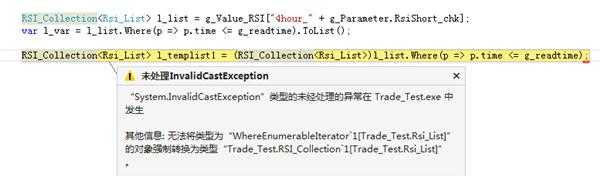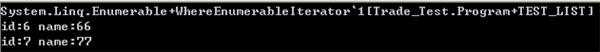﻿ LINQ 查询实体类的类型不符 | Code Bye

# LINQ 查询实体类的类型不符

7年前 (2016-09-18) 1185次浏览
PART_Collection<PART_List> l_list = g_Value[“AA” ];
PART_Collection<PART_List> l_templist = l_list.Where(p => p.time <= g_readtime).ToList();

10

5

PART_Collection 是什么东西，谁能看出来？

5

5

Quote: 引用:PART_Collection<PART_List> l_templist = l_list.Where(p => p.time <= g_readtime).ToList() as PART_Collection<PART_List> ;

5

```
RSI_Collection<Rsi_List> l_list = g_Value_RSI["AA"];
var l_var = l_list.Where(p => p.time <= g_readtime).ToList();
RSI_Collection<Rsi_List> l_templist1 = l_list.Where(p => p.time <= g_readtime);
```

5

`a = 1;`

5

Linq 查询结果跟你的自定义的那个类型，凭什么就兼容呢？你没有给出任何说明。

5

```            //TEST_Collection<TEST_LIST>
var l_temp = l_list.Where(p => p.id > 5);
Console.WriteLine(l_temp.GetType());
foreach (var x in l_temp) Console.WriteLine("id:{0} name:{1}", x.id, x.name);
```CodeBye 版权所有丨如未注明 , 均为原创丨本网站采用BY-NC-SA协议进行授权 , 转载请注明LINQ 查询实体类的类型不符

[1034331897@qq.com]